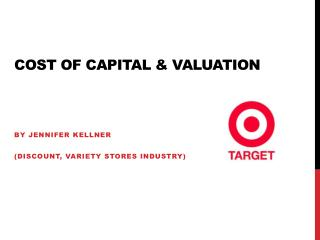DownloadDownload PresentationCost of Capital & valuation

# Cost of Capital & valuation

Télécharger la présentation## Cost of Capital & valuation

- - - - - - - - - - - - - - - - - - - - - - - - - - - E N D - - - - - - - - - - - - - - - - - - - - - - - - - - -
##### Presentation Transcript

1. Cost of Capital & valuation By jenniferkellner (Discount, Variety Stores Industry)

2. Discount, Variety stores industry Target Dollar General Wal-Mart Costco *Costco is least comparable

3. Objective • To calculate the Cost of Capital for Enterprise Operations at Target (WACC) • Using: • Cost of capital for equity (what investors will expect) • Investors expect to recover foregone interest and compensation for bearing the risk • Cost of capital for debt (rate of borrowing) • “The cost of capital represents a hurdle rate that a company must overcome before it can generate value” (Investopedia)

4. Steps • Calculate cost of debt capital • Locate market value of debt • Calculate cost of equity capital • Beta, regression, analysis • Calculate market value of equity • Finding WACC

5. Step 1: Cost of debt capital • Represents “The risk that Target will default on its debt” • 3 methods used: • FEAT/NFL • Weighted AvgInst Rate (from 2012 10K) x (1-t) • Interest expense/Avgamnt interest bearing debt

6. Cost of debt capital (cont) … • 1) 2) Relatively similar rates 3) • Since (1) uses reformulated f/s, which didn’t take into account much detail, more confidence in 2 & 3 • Take what Target says and what I recalculated, average the two:

7. Where the numbers come from: • Marginal Tax Rate: 2012 10K Note 23 • Weighted Avg Interest Rate: 2012 10K Note 20

8. Step 2: Market Value of debt 2012 10K (Note 9): • MV of Debt used: \$18,143 (million) • Close to 2012 NFL of \$17,648 • Chose to use Target’s number, when reformulating f/s did not take into account an abundance of detail. Target has thoroughly analyzed their debt to come up with this FV.

9. Step 3: Cost of equity • Inputs: • Beta • Alpha • Return on market

10. Beta • Beta: The extent to which the return on equity varies with the return on the market Conclusion: chose Bloomberg due to transparency in their calculations, wide use and reliability (also relatively close to other estimates, see above)

11. To demonstrate: regression Weekly: • Low Beta • Low R^2 • Other explanatory variables missing

12. Monthly: • Closer Beta to Bloomberg • Still low R^2 • Not using rf rate • Other explanatory variables ignored

13. Therefore, Cost of equity:

14. Step 4: Market value of equity • Closing price x Common shares outstanding • From Yahoo and 3rd Quarter 10Q (most recent data available)

15. Step 5: WACC Use equation and previous inputs (calculated) to determine return on the enterprise (WACC)

16. FCF Revisited • Using new WACC: 4.58% • Previously used: 6.08% (from Bloomberg) • Analysis: • Enterprise value changed significantly* • Prior E.V.: 94,432 • Why the difference? • Error • Discount rate sensitivity • Very close to sales growth (4.2%)

17. CAPM: An evaluation • + • Simplicity - Doesn’t account for additional risk factors (Ex: size of firm) • Beta: We assume immediate past is a suitable estimate for future risk • Stock based index (S&P500) doesn’t account for other investment opportunities *Conclusion: We should carefully consider the sensitivity of our valuation estimates to the cost of capital assumption

18. uncertainties • Is the model practical? To find WACC, we have to know the valuation in the first place (Mkt Value of equity, etc.) • *Beta is inevitably measured w/ error • Bloomberg • Return on market • *WACC and its inputs, requires significant assumptions

19. Important to note • The sensitivity of valuation to different WACCs (discount rates) • WACC based on many assumptions, can fluctuate greatly • Question reliability

20. Questions?